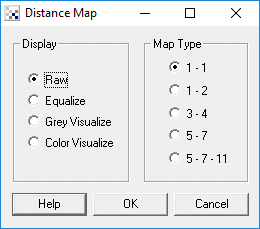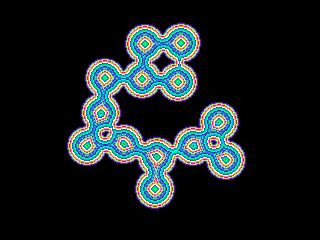# Distance Map

The Distance Map module performs a Euclidean distance map calculation. The distance map module replaces each foreground pixel with a number that represents the distance from that pixel to the border of the object. The distance map is useful in improving performance when calculating morphological operations such as erosion, dilation, etc.

## Interface## Instructions

1. Specify the how you would like to see the distance map.
Raw - display with actual calculated numbers.
Equalized - the raw numbers are spread across a 0-255 range to improve visibility of the different numbers.
Grey Visualize - the distance map bands are colored as grey values to better show the distance map elevations.
Color Visualize - like the Grey Visualize but with colored distance bands

2. Specify the map type the distance calculation should use. The map types relate to the approximated integer euclidean distances that each pixel represents. For example 1 - 2 represents the following map

```2 1 2
1 0 1
2 1 2
```

## Example

Source ImageColor Visualized Distance Map of 3 - 4 typeDistanceMap Related Forum Posts Last post Posts Views None